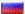# DYNAMIC CORRECTION OF ERRORS OF MEASURING CONVERTERS

doctor of science S.T. Tikhonchuk, A.V. Zadereyko

One of the biggest challenges in creating the automated control systems of complex objects and technological processes is the task of improving the accuracy of measurements.

When measuring fast processes should take into account the inertia of the components of the measuring channel. As a rule, the inertia measurement channel defined inertia sensor used. Confine correction measurements made using sensors, a mathematical model which can be represented as a delay element of the first, second or higher-order transfer function:

W(p) = 1/[(T1p+1)(T2p+1)*...*(Tnp+1)], n = 1,2,...,N (1)

A similar problem occurs whenever the rate of change of the measured parameter is comparable to the time constant of the sensor.

The basic approach to solving the problem of recovering the true parameter values at the input to output values measured approach is based on the construction of the converter transfer function implements the inverse of the transfer function of the sensor .

This converter is constructed by incorporating sensor model in a feedback channel operational amplifier with infinite gain. The transfer function Wk(p) = 1/W (p), as a rule, has a differentiating properties and attempts to build devices that implement this transfer function are generally not lead to success.

Correction algorithms implementing the transfer function Wk(p), obtained by unstable and moreover, significantly increase measurement errors.

Thus, the presence of measurement errors, which always have a place in the real measurement systems do not effectively implement this approach.

This problem can be partially solved by using the method of regularization. Discussed in relation to the problem of constructing devices for solving the inverse problem of correcting the measurements use the regularization method can be reduced to the use of an operational amplifier with a finite gain.

One possible solution to this problem is the approach that is implemented in , where due to the choice of structure has been avoided depending on the magnitude of the gain coefficient regularization.

The most promising approach to improve the accuracy characteristics of process control systems, is the use of single-device structures for dynamic correction of sensor errors.

## Fig.1. Functional diagram of the measurement path (+ sensor device dynamic correction)

Figure 1 shows a functional block diagram of the measurement path (sensor + dynamic correction device) with the resulting transfer function:

Wр(p) = W(p) * Wу(p) (2)

where W(p) - transfer function of the sensor (1) and Wy(p) - transfer function of the dynamic correction unit:

Wу(p) = 1/ [Wа(p) * К - К + Wc-1(p), (3)

where Wa(p) = W(p) - kernel driver with the transfer function is identical to the sensor.

K - static correction parameter, Wc(p) - a correction unit with a transfer function:

n=1

S hx px

x=0

Wc(p) = --------------- (4)

n

S fx px

x=1 x=1

Substituting (1) and (4) to (3) and without loss of generality: tending to the most simple form Wc(p), setting hx = 0 (x = 1, ... , n) on the basis of , we obtain:

h0*[(T1p+1)*...*(Tnp+1)]

Wу(p) = ----------------------------------------------------------------- (5)

n

Кh0 - Кh0 [(T1p+1)*...*(Tnp+1)][(Tnp+1)] * S fx px

x=1 x=1

The resulting transfer function of the entire measurement path described by the relation:

h0

Wу(p) = -------------------------------------------------------------------- (6)

n

Кh0 - Кh0 [(T1p+1)*...*(Tnp+1)][(Tnp+1)] * S fx px

x=1

We write the characteristic equation (6) as follows:

pn + A0 pn-1 + ... + An-1 p + An = 0, (7)

where A0, An-1, An - the coefficients of the corresponding powers of p of the characteristic equation of the transfer function (6).

To ensure the recovery of the measured signals with minimal distortion, it is necessary that the roots of the characteristic equation (7) were valid and had maximum modulus values , since the time constants of recovery signals are determined by the absolute values of the roots of pi, i = 1, ..., n the characteristic equation.